### Home > CAAC > Chapter 13 > Lesson 13.RE3-S > Problem2-150

2-150.
1. Decide whether each of the following pairs of expressions or equations are equivalent. If they are, show how you can be sure. If they are not, justify your reasoning completely. Homework Help ✎

1. (ab)2 and a2b2

2. 3x − 4y = 12 and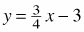3. y = 2(x − 1) + 3 and y = 2x + 1

4. (a + b)2 and a2 + b2

5.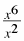and x3

6. y = 3(x − 5) + 2 and y = 2x −8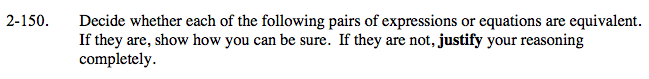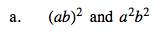Simplify the first expression.

(ab)2 = (ab)(ab) = a · a · b · b = a2b2

Substitute some numbers for a and b to confirm the algebraic manipulations you used are correct.

The first expression is equivalent to the second expression.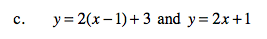See part (b).

Substitute some numbers for a and b to confirm the algebraic manipulations you used are correct.

The first equation is equivalent to the second equation.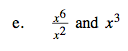Are these expressions equal for every value x?

This is an example of a place to investigate how using 0 gives a different result than other numbers.

The expressions are equivalent except when x = 0.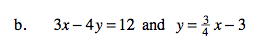Rewrite the first equation in y-form.

Substitute some numbers for a and b to confirm the algebraic manipulations you used are correct.

The first equation is equivalent to the second expression.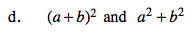Remember, (a + b)2 means to square the quantity a + b. You can use a generic rectangle to help you.

Substitute some numbers for a and b to confirm the algebraic manipulations you used are correct. Remember to be careful about using only 0 and 1. They can work and not work in very specific cases, often worth mentioning.

The first expression is NOT equivalent to the second expression.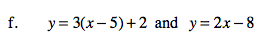Simplify the first equation.